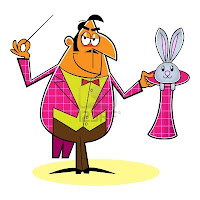## Sunday, December 23, 2012

### UR Love Magi ~ UR+L+M+

"Lovers don't finally meet somewhere.
They're in each other all along."
~ RUMI
RUMI = MI+UR = MIR+(7+7+7)
UR = 22+12+(2+0+1+2)
U+R = 21+18  = 39
21+18 = (7+7+7)+(6+6+6)
(2+2)+(1+2)+(2+0+1+2) = 12 = 3+9 = L
L. = Love
UR+L+M = 39+12+13 = 64 = 56+H

M. = Magi
1+6+2+6 = 3*5
1+6+26+64 = 33+64 = 97

"Of course there are robots among us.
There are also Magicians among us.
I think we take turns playing each role,
as a matter of fact.
The Magician defines a reality-mesh
and the robot lives in it.
Grok?"
~ Robert Anton Wilson = R.A.W.

I am Awake
R.A.W. & The Buddha
R+A+W+I+A = 52

B+S = 2+19 = 21 = 7+7+7
Robert Anton Wilson on B.S.
(Belief+Systems = B+S = Bull+Shit)
post scriptum:
12/12/12 Return Of The White Magi
&
The Covenant Of Love & Sound & Science
12/12/12 Al Kemet Gnosis Meditation:

I checked out of the lie they try to sell us every day
& prefer to look within my inner self
& I use the internet very often to gather information
& inspirations.

The Secrets of Kurt Gödel
22/42
The Secret History
"Life can be found only in the present moment.
The past is gone, the future is not yet here,
and if we do not go back to ourselves
in the present moment,
we cannot be in touch with life."
~ Thich Nhat Hanh

respectively:
EXorCist N°6*2 (E+X+C+N+6*2=32+N+12=58)
@ inner self infotainment (5+8)+E+(8+8+8)=42

"Whoever is careless with the truth in small matters cannot be trusted with important matters." ~ Albert Einstein

As Above, So Below

&
As Below, So Above...
O+T=35: Occult Triangulation

"Coincidence is what you have left over when you apply a bad theory." quote - Percy Williams Bridgman (Nobel Prize Winner in Physics & Philosopher of Science)

The Men You'll Never See ~ D.I.E. ~ 3:22
http://azurite888voyager.blogspot.de/2012/09/the-men-youll-never-see-detroit-in.html

“The way is not in the sky.

The way is in the heart.”
- Buddha (Siddhartha Gautama)

E.S.=Emerald Sword ~&&&~ E+S=D+A+D+O=X